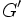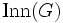# FZ implies finite derived subgroup

This article gives the statement and possibly, proof, of an implication relation between two group properties. That is, it states that every group satisfying the first group property (i.e., FZ-group) must also satisfy the second group property (i.e., group with finite derived subgroup)
View all group property implications | View all group property non-implications
Get more facts about FZ-group|Get more facts about group with finite derived subgroup

This result was proved by Schur and is sometimes termed the Schur-Baer theorem.

## Statement

### Verbal statement

If the inner automorphism group (viz the quotient by the center) of a group is finite, so is the derived subgroup. In fact, there is an explicit bound on the size of the commutator subgroup as a function of the size of the inner automorphism group.

### Symbolic statement

Let$G$ be a group such that$\operatorname{Inn}(G) = G/Z(G)$ is finite. Then,$G' = [G,G]$ is also finite. In fact, if$|G/Z(G)| = n$, then$G'$ has size at most$n^{2n^3}$.

### Variety-theoretic statement

The variety of abelian groups is a Schur-Baer variety.

## Facts used

1. Exterior square of finite group is finite
2. Commutator map is homomorphism from exterior square to derived subgroup of central extension (and this homomorphism is surjective by definition)

## Proof

### Proof using the alternate formulation

Given: A group$G$ such that$\operatorname{Inn}(G)$ is finite.

To prove:$G'$ is finite and its order is bounded in terms of the order of$\operatorname{Inn}(G)$.

Proof:

Fact no. Assertion/construction Facts used Given data used Previous steps used Explanation
1 There is a surjective homomorphism$\operatorname{Inn}(G) \wedge \operatorname{Inn}(G) \to G'$ (here,$\operatorname{Inn}(G) \wedge \operatorname{Inn}(G)$ denotes the exterior square of$\operatorname{Inn}(G)$). Fact (2)$G$ can be viewed as a central extension with quotient group$\operatorname{Inn}(G)$ (i.e., there is a short exact sequence$0 \to Z(G) \to G \to \operatorname{Inn}(G) \to 1$) and we can then apply Fact (2).
2 The group$\operatorname{Inn}(G) \wedge \operatorname{Inn}(G)$ is finite. Fact (1)$\operatorname{Inn}(G)$ is finite. fact-given combination direct.
3 The group$G'$ is finite and its order is bounded in terms of the order of$\operatorname{Inn}(G)$. The image of a finite group under a homomorphism is finite. Steps (1), (2) Fact-given direct. Note that the order being bounded follows from the fact that it is boudned by the order of$\operatorname{Inn}(G) \wedge \operatorname{Inn}(G)$. Since there are only finitely many isomorphism types of$\operatorname{Inn}(G)$ for a given order$n$, we can take the maximum over all these of the orders of their exterior squares, thereby getting a finite bound, in terms of$n$, on the orders of these. Explicit versions of the bound on the size in Fact (1) allow explicit bounds here.

### Direct proof outline

The proof involves two steps:

• Showing that the number of distinct commutators is at most$n^2$: This follows from the fact that the commutator$[x,y]$ depends only on the quotients of$x$ and$y$ modulo$Z(G)$, and thus there are$n^2$ possibilities.
• Showing that for any element in the commutator subgroup, there is a minimal word for that element with each commutator occuring at most$n$ times: This shows that any element of the commutator subgroup has a word in terms of the commutators, of length at most$n^3$, and this completes the proof.